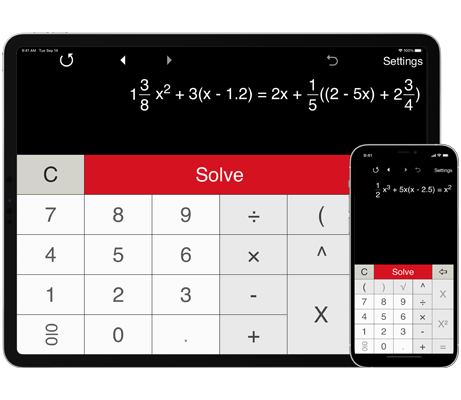# Quadratic equation solver with steps

## Solving quadratic equations step by step

This handy calculator can solve a wide range of quadratic equations (second degree equations), including quadratic equations containing fractions and parentheses. Note: Quadratic equation solver does not solve equations with a variable in the denominator.

It solves equations using the quadratic formula and shows the step by step solution.

Quadratic equation solver provides three pre-defined formats for solving quadratic equations in the form:
ax2 + bx + c = 0, ax2 + bx = 0 and ax2 + c = 0. The coefficients could be integers, decimals and fractions.

You can view calculation history and recall previous equations.

Quadratic equations solver lets you solve equations with real and complex roots.